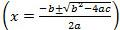# Let's Get Started

We're going to learn the steps to solving a quadratic equation by factoring, completing the square, and using the quadratic formula.  You will be able to solve problems using all three of these methods.

TEKS Standards and Student Expectations

A(8) Quadratic functions and equations. The student applies the mathematical process standards to solve, with and without technology, quadratic equations and evaluate the reasonableness of their solutions. The student formulates statistical relationships and evaluates their reasonableness based on real-world data. The student is expected to:

A(8)(A) solve quadratic equations having real solutions by factoring, taking square roots, completing the square, and applying the quadratic formula

Resource Objective(s)

Given a quadratic equation, the student will solve the equation by factoring, completing the square, or by using the quadratic formula.

Essential Questions

Why does factoring help us solve a quadratic equation?

When do we use completing the square to solve an equation?

What is the quadratic function? And how do you know the values of a, b, and c?

Vocabulary

# Solve Quadratic Equations by Factoring

Video

Watch the following video to learn how to solve a quadratic equation by using factoring.

Source

Practice

Select the steps on the right and drag them to their appropriate position to show the correct progression in steps to solve the quadratic equation when factoring 2x² - 5x = 12.

# Solve Quadratic Equations by Completing the Square

Video

Watch the following video to see how to solve a quadratic equation by completing the square.

Source

Practice

Select the steps on the right and drag them to their appropriate position to show the correct progression in steps to solve the quadratic equation x² - 20x = -51 by completing the square.

Your turn: Solve the quadratic equation x² + 12x − 28 = 0 by completing the square.

Click on the hints for help, the solution for the answer, and the detailed solution to explain the steps.

Video

Watch the following video to learn how to solve quadratic equations using the quadratic formula.

Source

Practice

Put the following steps in the correct order to solve the equation below using the quadratic formula.